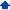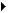### Creating two-variable What-If tables

Two-variable What-If tables show the results of varying two values in a What-If formula. Unlike one-variable tables, you can use only one formula. You can create a basic table that uses only the data you supply to calculate values, or you can build a table that refers to data already entered in the notebook database.

For example, suppose you own a health club and want to try a sliding-scale fee schedule. You devise a formula to calculate individual yearly fees:

`  Family Income - (No. of Dependents * 1000)/100   `

Insert graphic WhatIf3 A sliding scale lookup table. 1) Column Input Cell 2) Salary 3) Row Input Cell 4) Number of Dependents.

Now you want to build a lookup table to make it easier to pinpoint fees. The next figure displays a sample What-If table using this formula:

You can build similar tables showing all kinds of information — for example, a mileage chart listing miles per gallon for different amounts of gas used and numbers of miles driven, or profit amounts for a range of prices and costs.

##### Using two-variable What-If tables with a notebook database

A two-variable What-If table can draw out and analyze information in a notebook database. When you create this kind of table, you can use database @ functions to calculate values.

The following example of an employee notebook database uses a What-If table to show how long people have been employed in each department. A criteria table (F12..G13) is set up for two fields: Dept. and Years. The empty cells below the field headings are the input cells. The first column of the data table (F3..F6) lists each department. The first row (G2..I2) shows a range of years employed. The formula in cell F2 counts the number of employees for each department and employment length that’s shown in the criteria table:

What-If table showing employment lengths by department. 1) Years employed 2) Salary 3) Department 4) Employee name 5) Length of employment table 6) Formula used in the table 7) Criteria table uses F13 as the column input cell and G13 as the row input c,WhatIf4

@DCOUNT(A3..D16, 0,F12..G13)

#### To create a two-variable What-If table1 Type a column of substitution values.
 2 Starting in the row above and to the right of the substitution column, type a second column of substitution values.
 3 In the top-left cell of the table, type the formula to use to calculate table values.
 The formula must refer to two blank cells.
 4 Click ToolsNumeric toolsWhat-If tables.
 5 Enable the Two free variables option.
 6 Click the Range pickerin the What-If table box, and select the cells containing both formulas and substitution values.
 7 Click the Range pickerin the Column input cell box, and select the column input cell referenced in the What-If formula.
 8 Click the Range pickerin the Row input cell box, and select the row input cell referenced in the What-If formula.
 9 Click Generate.

#### To use a two-variable table with a notebook database1 Click ToolsNumeric toolsWhat-If tables.
 2 Enable the Two free variables option.
 3 Click the Range pickerin the What-If table box, and select the cells containing both formulas and substitution values.
 4 Click the Range pickerin the Column input cell box, and select the column input cell referenced in the What-If formula.
 5 Click the Range pickerin the Row input cell box, and select the row input cell referenced in the What-If formula.
 6 Click Generate.